◀123456789101112131415 본 문서의미리보기는15 Pg 까지만가능합니다. ▶
더크게 보기 ▼
닫기 ▲
•1

•2

•3

•4

•5

•6

•7

•8

•9

•10

•  본 문서의(큰 이미지) 미리보기는10 Page 까지만가능합니다.
작게 보기 ▲

# kenneth A.로스 (Ross) 해석학 솔루션 입니다 7장~36장

 솔루션 > 기타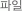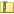kenneth A.Ross 해석학 솔루션입니다 7장~36장.zip   [Size : 2 Mbyte ]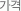1,500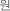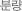99 Page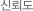94%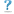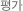자료설명

## HWP 파일로 되있구요.7장 ~ 36장까지 있습니다.미리보기 확인하시고 다운 바랍니다.

본문/목차

### Exercises 1 1. Prove that for all natural numbers . proof) Let . Since , is true. Suppose that is true. i.e. So, is true. By mathematical Induction, is true for all natural numbers . 2. Prove for all natural numbers . proof) Let . Since , is true. Suppose that is true. i.e. So, is true. By mathematical Induction, is true for all natural numbers . 3. Prove for all natural numbers . proof) Let . Since , is true. Suppose that is true. i.e. So, is true. By mathematical Induction, is true for all natural numbers . 4. (a) Guess a formula for by evaluating the sum for and . [For , the sum is simply .] sol.) So, we guess . (b) Prove your formula using mathematical induction. proof) Let . Since , is true. Suppose that is true. i.e. So, is true. By mathematical Induction, is true for all natural n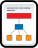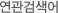해석학   kenneth   로스   Ross   입니다   7장~36장

 회사소개 | 개인정보취급방침 | 고객센터 ㅣ olle@olleSoft.co.kr 올레소프트 | 사업자 : 408-04-51642 ㅣ 광주 서구 상무민주로 104, 106-2002 | 채희준 | 통신 : 서구272호 Copyrightⓒ www.allreport.co.kr All rights reserved | Tel.070-8744-9518
개인정보취급방침고객센터olle@olleSoft.co.kr 올레소프트 | 사업자 : 408-04-51642 | Tel.070-8744-9518